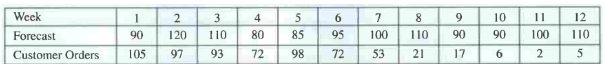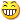## Case Study : Acme Water Pumps

The Acme Water Pump company has a problem. The pumps are fairly expensive to make and store, so the company tends to keep the inventory low. At the same time, it is important to respond to demands quickly, since a customer who wants a water pump is very likely to get one from a competitor if Acme doesn’t have one available immediately. A cm e’s current policy to produce pumps is to produce 100 per week, which is the average demand. Even this is a problem, as the production manager has pointed out, since the equipment is also used for other products and the lot size of 300 would be much more efficient. He said he is currently set up for water pump production for the next week and states that he has capacity available to produce 300 at a time next week.

The following lists the forecasts and actual customer orders for the next 12 weeksThe president of Acme has said that he wants to consider using a formal MPS with ATP logic to try to meet demand more effectively without a large impact on inventory. Acme has decided to use a demand time fence at the end of week 3 and has also found out that its current inventory is 25 units. Assume Acme will use the MPS lot size of 300 and that it will produce the first of those lots in week 1.

Assignment

1. Develop a master schedule using the information above.
2. A customer has just requested a major order of 45 pumps for delivery in week 5. What would you tell the customer about having such an order? Why? What, if any­ thing, would such an order do to the operation?

• Keep inventory low
• Respond demand quickly
• currently produce 100 pumps a week.
• Setting up 300 pumps a week
• Current inventory is 25 units.
• Use MPS lot size of 300 (first week)

1. Master schedule

Current inventory is 25 units.

 No Period A B C D E F G H I J K L 1 1 2 3 4 5 6 7 8 9 10 11 12 2 Forecast 90 120 110 80 85 95 100 110 90 90 100 110 3 Cust. orders 105 97 93 72 98 72 53 21 17 6 2 5 4 Projected Available Balance 220 123 30 250 152 57 257 147 57 267 167 57 5 MPS 300 300 300 300 6 ATP 30 58 209 287

Formula Projected Available Balance :

A4 = A5+25-A3 =  300 + 25 – 105 = 220

B4 = A4-B3 =  220 – 97 = 123

C4 = B4-C3 =  123 – 93= 30

D4 = D5+C4-MAX(D23) =  300 + 30 – MAX(80:73) = 250

E4 = D4-MAX(E2:E3) =  250 – MAX(85:98) = 152

F4 = E4-MAX(F2:F3) =  152 – MAX(95:72) = 57

G4 = G5+F4-MAX(G2:G3) =  300 + 57 – MAX(100:53) = 257

H4 = G4-MAX(H2:H3) =  257 – MAX(110:21) = 147

I4 = H4-MAX(I2:I3) =  147 – MAX(90:17) = 57

J4 =  J5+I4-MAX(J2:J3) =  300 + 57 – MAX(90:6) = 267

K4 =  J5-MAX(K2:K3) =  267 – MAX(100:2) = 167

L4 =  K4-MAX(L2:L3) =  167 – MAX(110:5) = 57

Formula ATP:

A6 =A5+25-SUM(A3:C3) = 300 + 25 – SUM(A3:C3) = 30

D6 = D5-SUM(D3:F3) = 300 – SUM(D3:F3) = 58

G6 = G6-SUM(G3:I3) = 300 – SUM(G3:I3) = 209

J6 = J6-SUM(J3:L3) = 300 – SUM(J3:L3) = 287

2. Request of 45 pumps

Cust. orders week 5 = 98 + 45 = 143

 Period 0 1 2 3 4 5 6 7 8 9 10 11 12 Forecast 90 120 110 80 85 95 100 110 90 90 100 110 Cust. orders 105 97 93 72 143 72 53 21 17 6 2 5 PAB 25 220 123 30 250 107 12 212 102 12 222 122 12 ATP 30 0 0 13 0 0 209 0 0 287 0 0 MPS 300 0 0 300 0 0 300 0 0 300 0 0

Berdasarkan penghitungan di atas, tambahan permintaan 45 pump pada minggu ke 5 tidak berdampak pada kekurangan persediaan pada minggu-minggu berikutnya. Sehingga, tidak perlu adanya penggunaan produk yang dialokasikan dari ATP pada minggu sebelumnya. Permintaan tersebut dapat diterima tanpa perlu ada penambahan proses operasi pada produksi.

Dilihat sebanyak : 463 kali

This site uses Akismet to reduce spam. Learn how your comment data is processed.